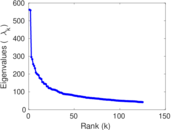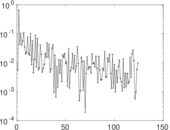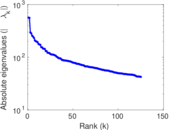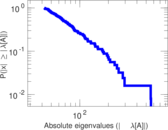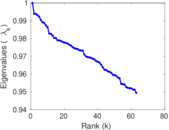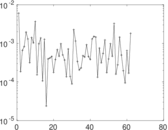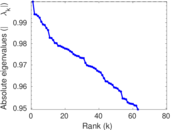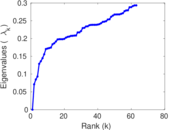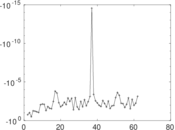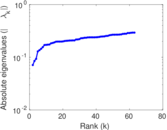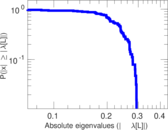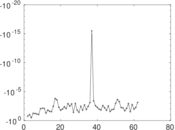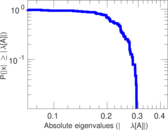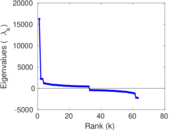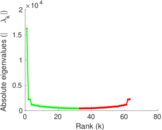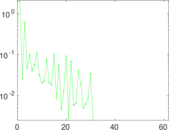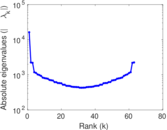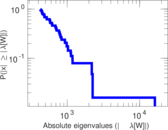# Wikiversity edits (es)

This is the bipartite edit network of the Spanish Wikiversity. It contains users and pages from the Spanish Wikiversity, connected by edit events. Each edge represents an edit. The dataset includes the timestamp of each edit.

 Code `yes` Internal name `edit-eswikiversity` Name Wikiversity edits (es) Data source http://dumps.wikimedia.org/ AvailabilityDataset is available for download Consistency checkDataset passed all tests Category Authorship network Dataset timestamp 2017-10-20 Node meaning User, article Edge meaning Edit Network formatBipartite, undirected Edge typeUnweighted, multiple edges Temporal dataEdges are annotated with timestamps

## Statistics

 Size n = 16,270 Left size n1 = 3,231 Right size n2 = 13,039 Volume m = 82,011 Unique edge count m̿ = 29,901 Wedge count s = 21,970,131 Claw count z = 24,887,184,101 Cross count x = 23,657,801,169,079 Square count q = 5,568,298 4-Tour count T4 = 132,513,186 Maximum degree dmax = 14,330 Maximum left degree d1max = 14,330 Maximum right degree d2max = 1,558 Average degree d = 10.081 3 Average left degree d1 = 25.382 5 Average right degree d2 = 6.289 67 Fill p = 0.000 709 748 Average edge multiplicity m̃ = 2.742 75 Size of LCC N = 15,240 Diameter δ = 11 50-Percentile effective diameter δ0.5 = 3.570 84 90-Percentile effective diameter δ0.9 = 5.206 26 Median distance δM = 4 Mean distance δm = 4.064 45 Gini coefficient G = 0.789 497 Balanced inequality ratio P = 0.177 537 Left balanced inequality ratio P1 = 0.119 386 Right balanced inequality ratio P2 = 0.236 956 Relative edge distribution entropy Her = 0.789 862 Power law exponent γ = 2.783 13 Tail power law exponent γt = 2.391 00 Tail power law exponent with p γ3 = 2.391 00 p-value p = 0.001 000 00 Left tail power law exponent with p γ3,1 = 1.981 00 Left p-value p1 = 0.096 000 0 Right tail power law exponent with p γ3,2 = 2.901 00 Right p-value p2 = 0.334 000 Degree assortativity ρ = −0.175 572 Degree assortativity p-value pρ = 1.369 21 × 10−205 Spectral norm α = 561.844 Algebraic connectivity a = 0.071 086 9 Spectral separation |λ1[A] / λ2[A]| = 1.005 65 Controllability C = 11,688 Relative controllability Cr = 0.723 178

## Plots

### Fruchterman–Reingold graph drawing### Degree distribution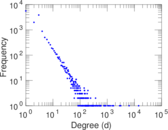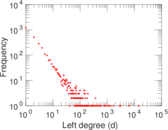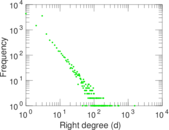### Cumulative degree distribution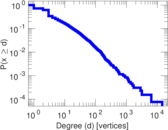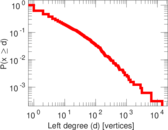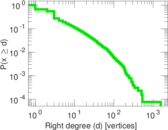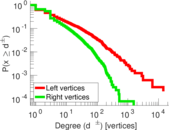### Lorenz curve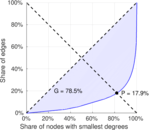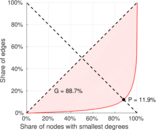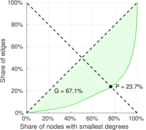### Spectral distribution of the adjacency matrix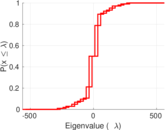### Spectral distribution of the normalized adjacency matrix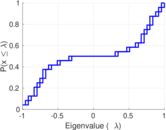### Spectral distribution of the Laplacian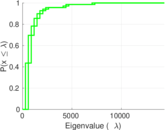### Spectral graph drawing based on the adjacency matrix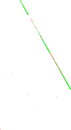### Spectral graph drawing based on the Laplacian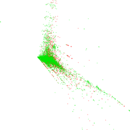### Spectral graph drawing based on the normalized adjacency matrix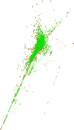### Degree assortativity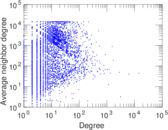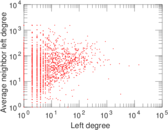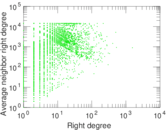### Zipf plot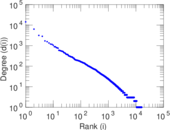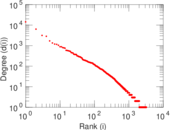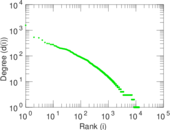### Hop distribution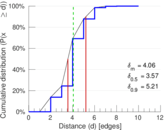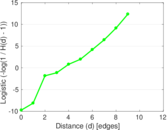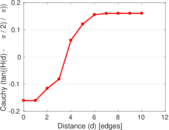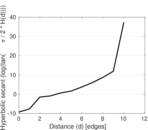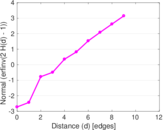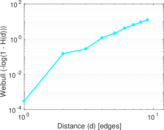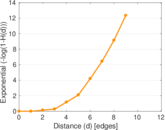### Double Laplacian graph drawing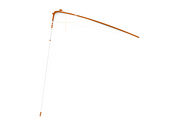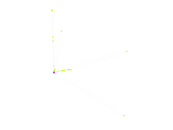### Delaunay graph drawing### Edge weight/multiplicity distribution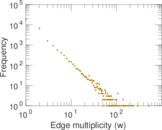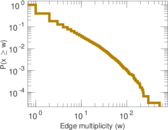### Temporal distribution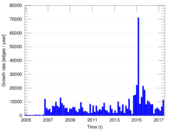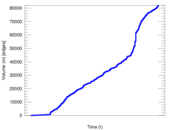### Temporal hop distribution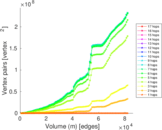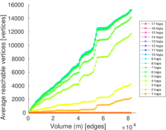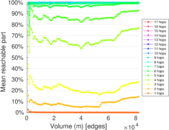### Diameter/density evolution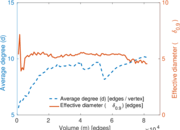### Matrix decompositions plots